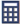This compound interest calculator calculates interest between any two dates. A dozen compounding frequencies are supported (did we miss any? :). You can also enter negative interest rates.

Because this calculator is date sensitive, and because it supports many compounding options, it is a suitable tool for calculating the compound interest owed on a debt when the debtor has not made any payments or from a point in time when the balance is known. More details below the calculator

×

#### Info...

Original Size

Click, copy, paste this URL to save the inputs for yourself or to share with others.

This custom URL updates when you click the "Calc" or "Clear" buttons. Paste it into a browser's address bar and press enter to reload.

## Compound Interest

Compound interest means that interest gets paid (or is earned) on previously paid interest.

For example, if the interest rate is 2% and you start with \$1,000 after the end of a year, you'll earn or owe \$20 in interest (using annual compounding). Then at the end of two years, assuming there have been no withdrawals (or payments) you earn \$20.40, not \$20. The previous interest earns interest as well.

This pattern is called compounding, and it repeats as long as the money stays invested, or the debt is owed.

If you are an investor, you want to compound interest. If you are a debtor, you want to avoid it, particularly if you ever miss a payment or a payment is not enough to cover the interest due.

You can use this online interest calculator as a:

• loan interest calculator
• savings interest calculator
• daily interest calculator
• negative interest calculator
• investment interest calculator

As a side benefit to this calculator's date accuracy, you can use it for date math calculations. That is, given two dates, it will calculate the number of days between them or it will find the date that is "X" days from the first date.Join the conversation. Tell me what you think.